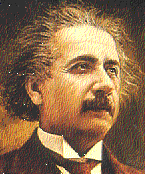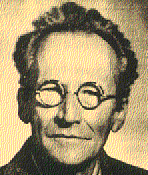The Einstein-Schrodinger theory without a cosmological constant was originally proposed by Einstein and Straus in 1946. In this form it is also known as "Einstein's Unified Field Theory", or the "Einstein-Straus Theory", or the "Hermitian Theory of Relativity", or the "Generalized Theory of Gravitation", or the "Relativistic Theory of the Non-symmetric Field". In 1947 Schrodinger showed that the theory can be derived from a very simple Lagrangian density if a cosmological constant is included. This more general theory is usually called "Schrodinger's Affine Field Theory" or the "Einstein-Schrodinger Theory". The Einstein-Schrodinger theory is a generalization of ordinary general relativity which allows a non-symmetric fundamental tensor. When Einstein and Schrodinger developed the theory they suspected that this fundamental tensor might contain both the metric and electromagnetic field, and they hoped that the theory would unify gravitation and electromagnetism. However, using the EIH method it was shown way back in 1953 that the original theory apparently does not predict a Lorentz force between charged particles. Since then there has been some interest in the theory by a few researchers who dispute the proof of the missing Lorentz force. Other researchers have assumed that the theory is correct but represents something other than gravity and electromagnetism. Still others have investigated theories which are significantly modified versions of the original theory. In general though, there has not been much interest in the Einstein-Schrodinger theory since the 1950s. Here are a few of Einstein's and Schrodinger's original papers on this topic.

Lambda-renormalized Einstein-Schrodinger theory:
Recently it has been shown that a well motivated modification of the Einstein-Schrodinger theory does indeed closely approximate ordinary general relativity and electromagnetism (Einstein-Maxwell theory), the modification being the addition of a large cosmological constant. This cosmological constant which multiplies the symmetric metric is assumed to be nearly cancelled by Schrodinger's ``bare'' cosmological constant which multiplies the nonsymmetric fundamental tensor, resulting in a total "physical" cosmological constant which matches measurement. The fact that these two cosmological constants multiply different fields has the effect of creating a Lorentz force, and also fixes some other problems with the original theory. One possible origin of the large cosmological constant is from zero-point fluctuations. It is reasonable to assume that the Einstein-Schrodinger theory must eventually be quantized to accurately predict reality, and this cosmological constant can be viewed as a kind of zeroth order quantization effect. The cancellation of cosmological constants resembles renormalization in quantum field theory, as in the cancellation of large particle ``self energies'' with large ``bare'' masses resulting in small ``physical'' masses. Another possible origin of the large cosmological constant is from a Higgs field vacuum expectation value as in GUT theories. The Lambda-renormalized Einstein-Schrodinger theory closely approximates Einstein-Maxwell theory regardless of the origin of the cosmological constant, and it becomes exactly Einstein-Maxwell theory in the limit as this cosmological constant goes to infinity. In addition, when the fields are generalized to be composed of Hermitian matrices, the theory closely approximates Einstein-Maxwell-Yang-Mills theory. This suggests several possibilities for a complete unified theory by using the Fermion and Higgs terms of GUT theories as sources.

For the details, see my papers:
"A modification of Einstein-Schrodinger theory that contains Einstein-Maxwell-Yang-Mills theory" (Gen. Rel. Grav. version),
"A modification of Einstein-Schrodinger theory that contains Einstein-Maxwell-Yang-Mills theory" (self-archived version),
"A modification of Einstein-Schrodinger theory that contains Einstein-Maxwell-Yang-Mills theory" (arxiv.org version),
"A modification of Einstein-Schrodinger theory that contains both general relativity and electrodynamics" (Gen. Rel. Grav. version),
"A modification of Einstein-Schrodinger theory that contains both general relativity and electrodynamics" (arxiv.org version),
"Einstein-Schrodinger theory in the presence of zero-point fluctuations",
"Einstein-Schrodinger theory using Newman-Penrose tetrad formalism",
"Lambda-Renormalized Einstein-Schrodinger theory with spin-0 and spin-1/2 sources".

Here are some of my presentations on this topic:
Shifflett_Dissertation_Presentation.ppt - given 22 April 2008 at Washington University,
Non-Abelian_LRES.pdf - given at the 2008 April APS meeting,
Einstein_Schrodinger.pdf - given at the 2007 April APS meeting,
shifflett_james_win.pdf - given 17 Nov 2006 at the 16th Midwest Relativity Meeting.

Here are some MAPLE programs associated with this theory.

Here is my dissertation:
Shifflett_James_A_Dissertation.pdf (self-archived version),